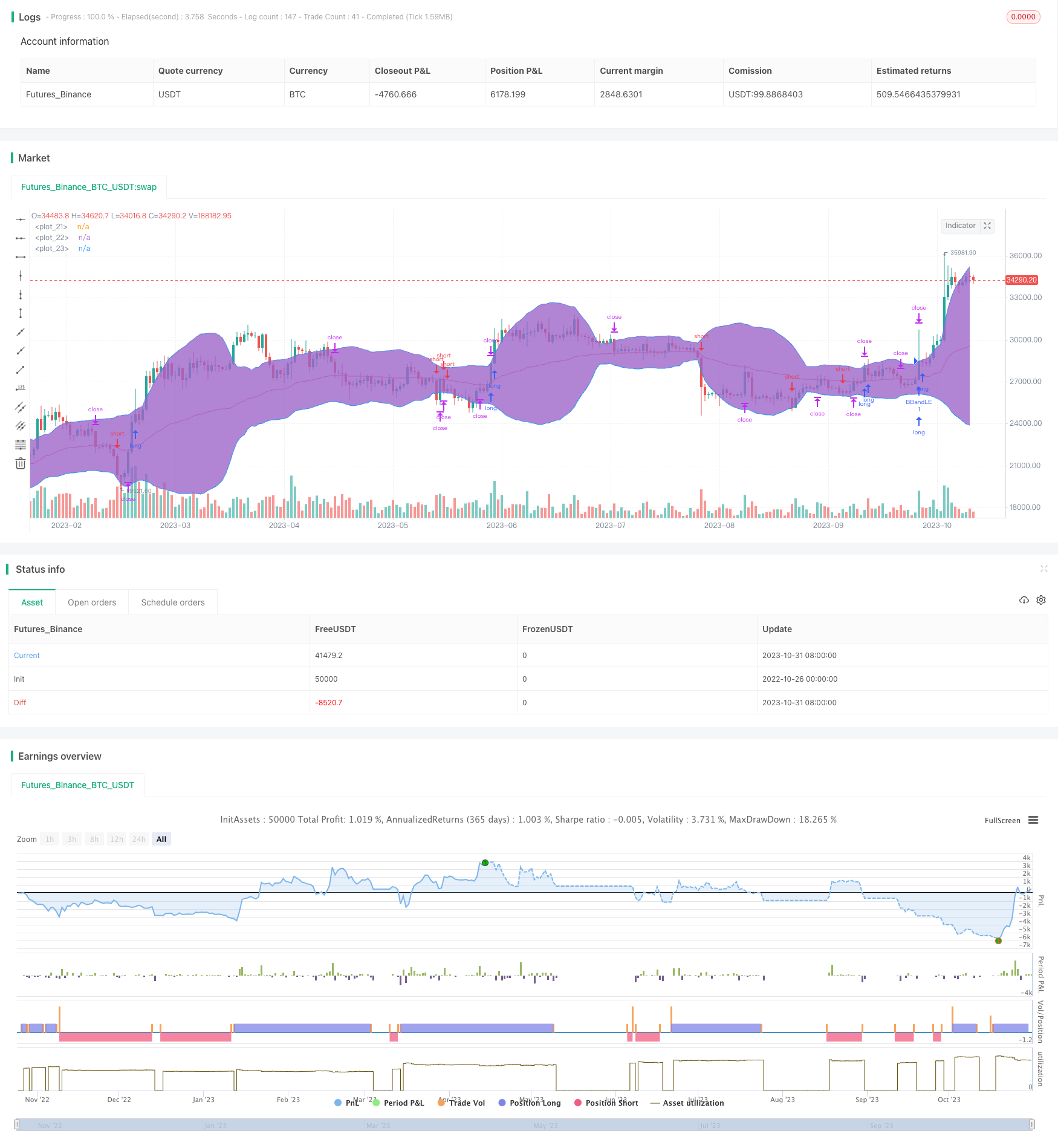# EVWBB Strategy Based on EVWMA and Bollinger Bands

Author: ChaoZhang, Date: 2023-11-02 15:27:28
Tags:## Overview

This strategy uses EVWMA as the basis line for Bollinger Bands. It goes long when the price breaks through the upper band and goes short when the price breaks through the lower band to capture trending moves in the price.

## Strategy Logic

The strategy first calculates the total volume over the past 30 periods as vol_period. Then it calculates EVWMA using the formula: (previous EVWMA x (vol_period - current volume) + current volume x close) / vol_period.

The basis for the Bollinger Bands is set as EVWMA, and the upper and lower bands are basis ± 2 * stdev(close). The strategy goes long when the price breaks above the upper band and goes short when the price breaks below the lower band. The stop loss is set at the basis level.

1. EVWMA reflects price changes better than moving averages, resulting in a smoother line.

2. Bollinger Bands clearly identify the upper and lower limits of price fluctuations, making it easy to capture breakouts.

3. Combining the trend indicator EVWMA and the volatility indicator Bollinger Bands allows more precise timing of entries.

4. The stop loss at the basis level helps control risk.

## Risk Analysis

1. EVWMA may fail to reflect price changes in time during huge market swings, causing missed entry opportunities.

2. Bollinger Bands are prone to whipsaws during range-bound markets, triggering unnecessary entries.

3. Lack of position sizing and holding period management can lead to unsatisfactory profits or magnified losses.

4. Absence of a profit target risks holding positions beyond reasonable objectives.

## Optimization Directions

1. Test different parameter settings to find optimal lookback periods.

2. Consider adding filters like MACD to refine entry signals.

3. Implement fixed holding period to manage trades.

4. Set profit targets to define reasonable profit goals.

5. Adjust position sizes based on market conditions.

## Summary

This strategy combines the strengths of EVWMA and Bollinger Bands to track trends by capturing breakouts. Its advantages are reasonable indicator combination, precise entries, and effective risk control. However, improper parameter tuning and lack of trade management remain issues. Further improvements in parameter optimization, profit targeting, stop losses, and position sizing can enhance its stability and profitability. Overall, the strategy logic is sound and shows practical value and development potential.

```/*backtest
start: 2022-10-26 00:00:00
end: 2023-11-01 00:00:00
period: 1d
basePeriod: 1h
exchanges: [{"eid":"Futures_Binance","currency":"BTC_USDT"}]
*/

//@version=4
strategy("EVWBB Strategy [QuantNomad]", shorttitle="EVWBB Strategy [QN]", overlay=true)

// Inputs
sum_length = input(30,  title = "Length", type = input.integer)
mult       = input(2.0, minval=0.001, maxval=50)

// Calculate Volume Period
vol_period = sum(volume, sum_length)

// Calculate EVWMA
evwma = 0.0
evwma := ((vol_period - volume) * nz(evwma, close) + volume * close) / (vol_period)

basis = evwma
dev = mult * stdev(close, sum_length)

upper = basis + dev
lower = basis - dev

plot(basis, color=color.red)
p1 = plot(upper, color=color.blue)
p2 = plot(lower, color=color.blue)
fill(p1, p2)